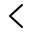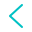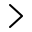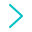Here I will try to show you some projects I’m pursuing

Lau
Some Examples
Dupin cyclides
A simple numerical approach.
- In Python -
Private Cloud
Using Seafile to create a private Cloud in the own livingroom.
Some Coding
Some little coding projects like calculating molar mass or processing scientific data.## Numerical approach to dupin cyclides

Dupin cyclides are geometric inversion of a standard torus or cylinder. They can be created by taking the hull of a set of spheres on an ellipsoid.
(Remark: Special case e.g. all the spheres have the same radius and the ellipse is a circle -> Torus)
I tried to write a little Python script for creating a plot for a specific type of dupin cyclides.

Some plots of dupin cyclides with different parameters.

For further information see for example Wikipedia or the University of Stuttgart for pictures of plaster models.

### Python code

Unfortunately this code takes quite some time for plotting (depending on the ‘resolution’ N) as I plot all spheres and not just the hull. For N=1000 a plotting time in the range of a few minutes is normal. For smaller N this can be reduced.

# -*- coding: utf-8 -*-
"""
Created on Sat Feb  8 18:57:35 2020

@author: Lau
"""

import numpy as np
import matplotlib.pyplot as plt
from mpl_toolkits.mplot3d import Axes3D
from timeit import default_timer as timer
from scipy.spatial import ConvexHull

class Zyklide:
def __init__(self, r_off=1.5, N=100, a=10, b=5):
self.N = N
self.a = a
self.b = b
r = np.cos(np.linspace(0, 2*np.pi, N))
self.r = r + r_off

def ellipse(self, a, b, N):
phi = np.linspace(0, 2*np.pi, N)
x = (a * np.cos(phi))
y = (b * np.sin(phi))
coords = np.array([x, y])
return coords

def sphere(self, x, y, r):
u, v = np.mgrid[0:2*np.pi:100j, 0:np.pi:100j]
x = r*np.cos(u)*np.sin(v)-x
y = r*np.sin(u)*np.sin(v)-y
z = r*np.cos(v)
return np.array([x, y, z])

def calculate(self):
start = timer()
self.ellips_coords = self.ellipse(self.a, self.b, self.N)
self.sphere_points = []
for i in range(self.ellips_coords.shape):
self.sphere_points.append(
self.sphere(self.ellips_coords[0, i],
self.ellips_coords[1, i],
self.r[i]))
end = timer()
print(f'Time for calculation: {end - start} s.')  # Time in seconds
return 0

def plot(self, theta=30, phi=45):
start = timer()
fig = plt.figure()
ax = Axes3D(fig)
ax.set_axis_off()
for i in range(self.ellips_coords.shape):
xi = self.sphere_points[i][0, :, :]
yi = self.sphere_points[i][1, :, :]
zi = self.sphere_points[i][2, :, :]
ax.plot_surface(xi, yi, zi, color='b')
ax.set_xlim(-10, 10)
ax.set_ylim(-10, 10)
ax.set_zlim(-10, 10)
ax.view_init(theta, phi)
plt.savefig('zyklide.png', dpi=600)
plt.show()
end = timer()
print(f'Time for plotting: {end - start} s.')  # Time in seconds

if __name__ == '__main__':
ring_zyklide = Zyklide(a=8,b=8,N=100)
ring_zyklide.calculate()
ring_zyklide.plot(theta=90, phi=0)


## Word Cloud – Bachelor Thesis

I also created a “word cloud” of my B.Sc. Thesis. My thesis has the title “Coupling into silicon waveguides” and was submitted to the Barz group at the Institute for Functional Matter and Quantum Technologies, University of Stuttgart.

### It’s very easy to create such a word cloud with python:

# -*- coding: utf-8 -*-
"""
Created on Wed Nov 13 16:00:23 2019

@author: Lau
"""

from wordcloud import WordCloud, STOPWORDS
import matplotlib.pyplot as plt

with open("Lau_BA.txt") as BA:

wordcloud = WordCloud(background_color='white',width=1920, height=1200,scale=1)
#STOPWORDS.update(['maybe', 'you', 'may', 'want', 'to', 'ignore', 'some', 'words'])
wordcloud.generate(text)
fig = plt.figure()
plt.imshow(wordcloud,interpolation="bilinear")
plt.axis('off')
plt.show()
wordcloud.to_file("WordCloud_Lau_BSc.pdf")

I just used a .txt file with the text from my thesis (CTRl+C & CTRL+V into an .txt ignores the figures) and loaded this plain text into the script.

## Molar Mass Calculator

I wrote a little program to calculate the molar mass of a compound. Furthermore it is able to calculate the required amount of reactants if you have a reaction formular.

You can get or review the program on GitHub:

https://github.com/LauKr/Molar-mass-calculator